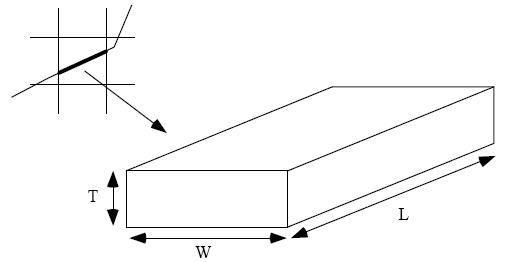# Fall Semester 2018

 Page 1 Page 2 Page 3 [Page 4] Page 5 Page 6

Essay Questions

Click here to access the Equation Sheet.

11. (modflow) For each of the following solvers variables, describe the effect (increase, decrease, no effect) that increasing the indicated variable has on the average time required for MODFLOW to converge to a solution.

a. Convergence tolerance (interval)

b. Max number of iterations

c. Acceleration parameter

12. (modflow) Define the term head overshoot as it relates to the solution process for MODFLOW models. Describe two things that can be done to minimize the odds of head overshoot occurring?

13. (calibration) What factors should be considered when determining a calibration interval (target) associated with a head observation at a monitoring well for use in calibrating a model. Explain (qualitatively) how the interval affects the overall model error measured by the sum of the squared weighted residuals.

14. (calibration) In the context of automated parameter estimation with pilot points, explain the concept of regularization. What is the main benefit of regularization? What are the two types of regularization?

15. (calibration) What is the difference between a forward run and an inverse run in the context of MODFLOW modeling?

16. (gms) The following diagram represents a section (reach) of a river that lies within a
MODFLOW cell:where L is the longitudinal direction of the river. Write the expression that would be used to compute the conductance factor that you would assign to a river arc in a GMS conceptual model associated with your MODFLOW model.

17. (calibration) Describe the two strategies we discussed for pilot point placement. What is the main advantage of each?